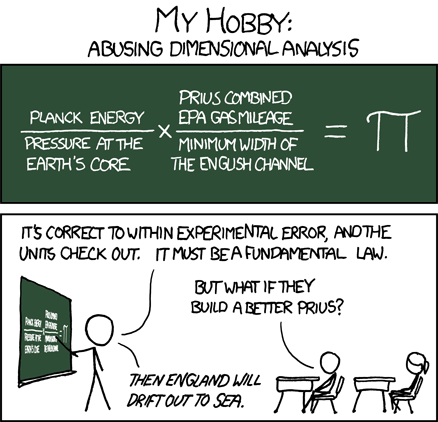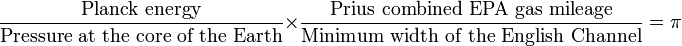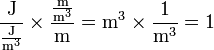# 687: Dimensional Analysis

 Dimensional AnalysisTitle text: Or the pressure at the Earth's core will rise slightly.

## Explanation

Cueball has a hobby — showing correct calculations according to the dimensional analysis — but with ridiculous correlations of uncorrelated events and measurements. Here Cueball is teaching a class and uses this trick to convince his students that the Toyota Prius combined EPA gas mileage is somehow connected to the constant π via the Planck energy, the pressure at the Earth's core and the width of the English Channel.

Scientists — often physicists — use dimensional analysis to quickly check if a given formula can possibly relate to a physical system, because if you end up with an equation claiming that joules are meters, something is clearly wrong. Dimensional analysis here refers to the check if both sides of the equation arrive at the same physical unit when the units of all variables get plugged into the equation. This requires knowledge of the system of units and the relation between different physical units.

Cueball uses the following equation to make a mockery of the practice:$\frac{\text{Planck energy}}{\text{Pressure at the core of the Earth}} \times \frac{\text{Prius combined EPA gas mileage}}{\text{Minimum width of the English Channel}} = \pi$

### Dimensional analysis

The right hand side is dimensionless, it's the constant π = 3.14... which is defined by the relation of two lengths, the circumference and the diameter of a circle. The left hand side requires to plug in the dimensions of the named physical quantities:

• Planck energy: given in Joules [J]
• Pressure at the core of the Earth: given in Pascals [Pa]
• Prius combined EPA gas mileage: miles per gallon, given in meters per cubic meter [m/m³]
• Fuel efficiency has two formats that are commonly used: length per volume and volume per length. The former must be used here in order to get the units to cancel correctly.
• Minimum width of the English Channel: given in meters [m]

When plugged into the left hand side this amounts to:$\frac{\text{J}}{\frac{\text{J}}{\text{m}^3}} \times \frac{\frac{\text{m}}{\text{m}^3}}{\text{m}} = \text{m}^3 \times \frac{1}{\text{m}^3} = 1$

Using the following unit relations (this does not reduce units to the seven SI base units, but does use some derived units for cancelation):

• 1 Pascal [Pa] = 1 Joule per cubic meter [J/m³]

Note that for dimensional analysis constant factors are not taken into account. Here square brackets are used to denote dimensional analysis. In the above equation the unit of energy (joule) as well as all the unit of volume (cubic meter) cancel out each other.

Another aspect of the comic is, that sometimes dimension analysis of equations that were not derived but rather "made up" can provide insight. However, in reality such an equations would have to be somehow "motivated", which is more of an art than science and requires great experience in the field the equation should relate to. The presented equation combines values that have no immediate causal relation with each other, so it does not make sense. Furthermore, since the values have absolutely no causal relation to each other, the ratios presented are simple coincidence; despite Cueball's claim, building a better Prius would not cause any changes to the English Channel.[citation needed]

The title text also refers to this, as a higher pressure at Earth's core could also balance the equation, keeping the result constant equal to π. The Planck energy is an absolute, however, so it is not mentioned as a way to balance the next version of Prius.

### Some numbers for this calculation

The Planck energy is the only nearly exact value we do have. Compared to other Planck values it is very large (macroscopic).

E_planck = 1.956 x 109 J


Pressure at the core of the Earth ranges from 330 to 360 gigapascals. Using a simple value like this:

P_core = 350 GPa = 3.5 x 1011 J/m³


Prius combined EPA gas mileage: For the third generation (from 2010) the City mileage is 51 mpg and the Highway mileage is 48 mpg. But it is the combined EPA gas mileage which is used in the equation and that is 50 miles per gallon.

50 mpg => 21,000,000 m/m³


Minimum width of the English Channel is about

33.1 km = 33,100 m


Calculating from these values you will get π = 3.54... that is pretty close to π = 3.14... while using a Planck value. According to Cueball this will be within the experimental error (the combined error for all four numbers - none are exact numbers). For instance if you tried the ePrius you would probably get closer to that target — as the mileage in real life usually is somewhat lower than the value given — and that would reduce the result.

## Transcript

My Hobby:
Abusing dimensional analysis
[On a blackboard.]
(Planck energy/Pressure at the Earth's core) x (Prius combined EPA gas mileage/Minimum width of the English Channel) = π
[Cueball indicates this equation with a pointer in front of a class.]
Cueball: It's correct to within experimental error, and the units check out. It must be a fundamental law.
Student: But what if they build a better Prius?
Cueball: Then England will drift out to sea.add a comment! ⋅add a topic (use sparingly)! ⋅refresh comments!

# Discussion

Made a minor addition to the explanation as it relates to the "Buckingham Pi" formalization. This may be a 2nd order pun in the comic.Tardyon (talk) 15:05, 3 February 2014 (UTC)

The units only check out if mileage is given in the form liters/100 km. If you use miles/gallon you end up with units of length^-4.108.162.217.5 22:17, 13 November 2014 (UTC)

Or, maybe the Plank Energy will decrease... Mountain Hikes (talk) 04:01, 17 December 2015 (UTC)

Why is he breaking from his usual black and white style? The green was a suprise 162.158.39.207 22:06, 27 November 2016 (UTC)Davy

England is part of the mainland of Britain and can't float anywhere on its own without tearing itself away from the rest of the land. It's like suggesting that California can float away from America. (Maybe that wasn't a good example, what with the San Andreas Fault, and all...) Brenda (talk) 09:52, 15 June 2018 (UTC)